Extraction of temperature dependences of small-signal model parameters in SiGe HBT HICUM model
Sun Ya-Bin1, Fu Jun2, †,, Wang Yu-Dong2, Zhou Wei2, Zhang Wei2, Liu Zhi-Hong2
College of Electronic Science and Engineering, Nanjing University of Posts and Telecommunications, Nanjing 210003, China
Tsinghua National Laboratory for Information and Technology, Institute of Microelectronics, Tsinghua University, Beijing 100084, China

† Corresponding author. E-mail: fujun@tsinghua.edu.cn

Project supported partially by the Important National Science & Technology Specific Projects, China (Grant No. 2013ZX02503003).

Abstract
Abstract

In this work, temperature dependences of small-signal model parameters in the SiGe HBT HICUM model are presented. Electrical elements in the small-signal equivalent circuit are first extracted at each temperature, then the temperature dependences are determined by the series of extracted temperature coefficients, based on the established temperature formulas for corresponding model parameters. The proposed method is validated by a 1 × 0.2 × 16 μm2 SiGe HBT over a wide temperature range (from 218 K to 473 K), and good matching is obtained between the extracted and modeled results. Therefore, we believe that the proposed extraction flow of model parameter temperature dependence is reliable for characterizing the transistor performance and guiding the circuit design over a wide temperature range.

1. Introduction

Because of its excellent performance and compatibility with conventional Si CMOS processing, SiGe HBT technology has recently received a great deal of attention in radio frequency (RF), microwave and extreme environment applications.[1,2] Since the existence of bandgap-engineering in the base region, the narrowed-bandgap induced by the Ge profile retards the classical problems associated with cooling conventional transistors, such as base freezeout, carrier diffusivity and mobility degradation. As a consequence, nearly all the transistor metrics improve with temperature decreasing, which enables SiGe HBT to operate well in a low temperature range. Obviously, on the other hand, SiGe HBTs also suffer some degradations in AC and DC characteristics as temperature increases.[3,6,7] However, it is lucky to find that the speed of such a degradation is not very fast. For example, even at 300 °C IBM the 2nd generation SiGe HBT also possesses a current gain of above 100 and fT above 75 GHz. It demonstrates a potential of SiGe HBT operating over a wide range of temperature. Therefore, it is vital to investigate the physics of SiGe HBT model parameters over a wide temperature, and also important to build the temperature dependences of model parameters with good fidelity.

A completed transistor model generally includes two parts: the small-signal model and the large-signal model. The small-signal model is a linear approximation of the large-signal model in a given bias condition and can accurately describe the transistor characteristics. The extraction of small-signal model parameters is less complicated, and it is promising to obtain the large-signal model parameters. Therefore, the temperature dependences of model parameters related to the small-signal equivalent circuit are presented in this work. The small-signal electrical elements in the SiGe HBT HICUM model in off-state are first extracted at a given temperature, and the corresponding temperature coefficients for each model parameter are then obtained based on the proposed temperature formulas. This paper provides a theoretical guide for designing the device and circuit of SiGe HBTs working in a wide temperature range.

2. Experiment

After the bias-independent extrinsic parasitic elements are removed through a standard ‘open’ and ‘short’ de-embedding pattern, the small-signal equivalent circuit of the SiGe HBT HICUM model under cut-off mode is obtained, as shown in Fig. 1. The temperature dependences of resistance and capacitance originate from the physical quantities such as the intrinsic carrier density, diffusivity or mobility. The temperature coefficients related to the model parameters in Fig. 1 are respectively the effective bandgap voltages VgEeff, VgCeff, and VgSeff, temperature exponents ζRB for base resistance, ζRCX for collector resistance, ζRSU for substrate resistance, and ζRE for emitter resistance.

 Figure Option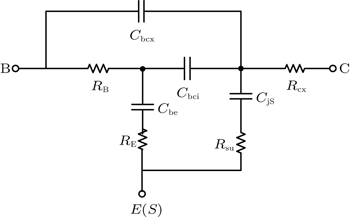Fig. 1. Simplified small-signal equivalent circuit in SiGe HBT HICUM model biased at off-state.

The devices under test are vertical NPN SiGe HBTs and five temperature points 218 K, 248 K, 298 K, 373 K, and 473 K are adopted. DC characteristics including the forward-Gummel and RC_flyback are measured with an Agilent B1500 Semiconductor Parameter Analyzer, and the S-parameter measurements are carried out in a microwave probing system using a vector network analyzer over a frequency range of 100 MHz–25 GHz.

3. Extraction of electrical elements

The junction capacitances are first extracted from the cold S-parameters measurement. For the BE junction capacitance Cbe and BC junction capacitance Cbc, base voltage VB is swept from −1 V to 0.4 V under a fixed VC = 0 V. Similarly, for the CS junction capacitance CjS, VC is swept from −0.4 V to 1.5 V with a constant VB = VS = 0 V. Then the junction capacitance can be directly determined from the following non-linear rational function fitting over the whole frequency range: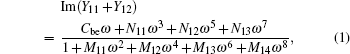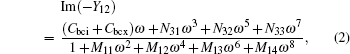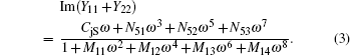The coefficients Ni j and Mi j of other high-order terms each are a function of the circuit elements in Fig. 1. Figure 2 shows the variations of Im(Y11 + Y12), Im(−Y12), and Im(Y22 + Y12) with angular frequency ω for 1 × 0.2 × 16 μm2 SiGe HBT biased at VB = VC = 0 V at room temperature. Excellent agreement is found over the whole frequency range, and the junction capacitances are respectively obtained to be 55.64 fF (55.04 fF, 56.24 fF) for Cbe, 62.78 fF (61.95 fF, 63.21 fF) for Cbcx + Cbci, and 26.10 fF (25.92 fF, 26.28 fF) for Cts. Confidence intervals (included in the above brackets) are narrow and hence the extracted junction capacitances are credibly accurate. For each operation point and measurement temperature, an appropriate fitting frequency range is adopted to obtain the global junction capacitance. As a consequence, the bias-dependent junction capacitances at different temperatures are determined, then the junction capacitance model parameters, zero-biased depletion capacitances, grading coefficients, and built-in voltage are obtained, which will be discussed in the following section.

 Figure OptionFig. 2. Comparison between the measured (symbols) and fitting results (solid lines) of formulas (1)–(3) for 1 × 0.2 × 16 μm2 SiGe HBT biased at VB = VC = 0 V at room temperature.

The substrate resistance RSU can be extracted by using the reported cold S-parameter approach. The external collector resistance RCX can be determined through the convenient flyback method. As for the emitter resistance RE, a novel extraction method has been proposed based on forward-Gummel measurements in our pervious study. In the medium current region we can obtain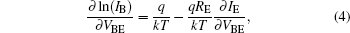where VBE represents the applied voltage across the BE junction, kT/q is the thermal voltage at given temperature T, IB, and IE are base current and emitter current in the forward Gummel measurement, RE can be determined from the least squares linear fitting of Eq. (4) when plotting ln(IB)/∂VBE versus ∂IE/∂VBE, as shown in Fig. 3, from which an almost straight line is expected in the medium current region for a 1 × 0.2 × 16 μm2 SiGe HBT at room temperature. It is demonstrated that expression (4) is reasonable and the extracted RE values are reliable. The deviation between the measured and fitted data in the high current region is due to the high injection and quasi-saturation effects. For example, the heterojunction barrier effect (HBE) and self-heating effect will lead to a slower increasing speed of IC (or IE) than that of IB with VBE increasing, thus a large deviation appears in the plot of the high current region, as shown in Fig. 3. Therefore, the proposed method avoids some losses of extraction precision induced by the high current effect.

 Figure Option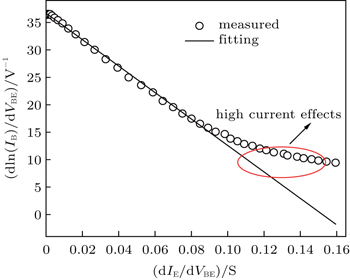Fig. 3. Typical fitting result of Eq. (4) for a 1 × 0.2 × 16 μm2 SiGe HBT.

As in our pervious study, the remaining model parameter RB can be obtained based on the nonlinear rational function fitting. Taking a 4 × 1.2 × 20 μm2 SiGe HBT under off-states for example, as shown in Fig. 4, RB and RCX both decrease with the external BC junction Cbcx increasing. RB is confined within a narrow range from 9.88 Ω to 10.59 Ω when RCX drops to zero. Then with the aid of pre-determined RCX of 4.18 Ω (already extracted from RC_flyback method), RB is obtained to be 10.17 Ω. In other words, the base resistance here is directly extracted from cold S-parameters without making any numerical optimization.

 Figure Option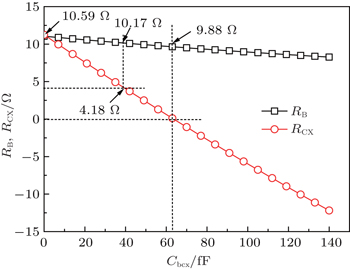Fig. 4. Plots of RB and RCX versus Cbcx for 4 × 1.2 × 20 μm2 SiGe HBT in cut-off state.

Through the given method as discussed above, the series resistances RCX, RSU, RE, and RB at each temperature can be extracted and the plots will be given below. By far, the problem of extracting each electrical element in the small-signal equivalent-circuit as shown in Fig. 1 has been completed. Then based on these extracted results at each temperature, the temperature dependence can be established, which will be discussed in the following section.

4. Temperature dependence of model parameters

In the HICUM model, the temperature dependence of model parameters is described via the physical quantities such as intrinsic carrier density, diffusivity or mobility. The temperature dependence of internal base resistance RB here depends on the carrier mobility in the neutral region. Then zero-bias resistance is described as: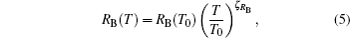where T0 is the reference temperature and RB(T0) is the determined base resistance at given temperature T0. The temperature coefficient ζRB is a function of base doping concentration. The external collector resistance RCX, emitter resistance RE, and substrate resistance RSU each follow a similar relationship to that shown in Eq. (5). The temperature coefficients ζRCX, ζBE, and ζRSU each are a function of average doping concentration in a corresponding region.

As in Ref. , all junction depletion capacitances are modeled in terms of the capacitance at zero bias Cj0, a built-in voltage VD and a grading coefficient zj. In HICUM the grading coefficient zj is assumed to be a constant and temperature-independent. To access the temperature dependence of built-in voltage VD, an auxiliary voltage VDj(T0) at given reference temperature T0 is calculated as indicated in Ref.  and given as follows: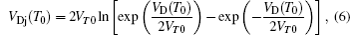where VD(T0) and VT0 are the built-in voltage and thermal voltage at reference temperature T0 respectively. With the linear temperature-dependent effective bandgap, the value VDj(T) at an arbitrary temperature is written as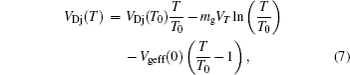where mg is a constant to characterize the temperature dependence of the intrinsic carrier density and Vgeff(0) is the finite bandgap at T = 0 K. Finally, the built-in voltage VD(T) can be calculated as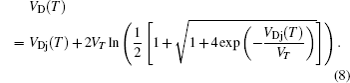It is noted that the built-in voltage VD is associated with the relevant junction region, for example, Vde is related to the base and emitter region, therefore Vgeff here should be an average effective bandgap, which is written as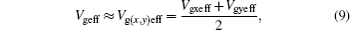with (x,y) = (B, E), (B, C), (C, S). Generally, the depletion junction Cj0 at zero bias is expressed as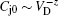, therefore, the temperature dependence of Cj0 can be directly obtained from the previously determined VD, and expressed as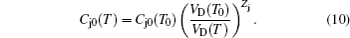The grading coefficient z here does not depend on temperature. As reported in Ref. , the well-known junction depletion capacitances can be modeled, respectively, as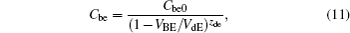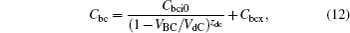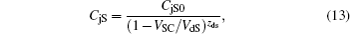where Cbe0, Cbci0, CjS0 are the zero-biased depletion capacitances; Zde, Zdc, ZdS are the grading coefficients; VdE, VdC, and VdS are the built-in voltages of BE junction, BC junction, and CS junction, respectively; and VBE, VBC, and VSC are the applied forward bias voltage across the junctions. Here the external BC junction Cbcx is assumed to be bias-independent.

It is noted that the built-in voltage VD is temperature dependent and the grading coefficient Zd is temperature-independent in the HICUM model, therefore, VD cannot be set as a constant similar to the case at room temperature. A novel parameter extraction flow for the depletion capacitances is recommend. First, the CV characteristics at given reference temperature T0 is fitted directly, then the built-in voltage VD, zero-biased capacitance Cj0 and grading coefficient Zd will be obtained simultaneously if high accuracy exists. Otherwise, set VD as the default value, and extract the Cj0 and Zd from the CV curve fitting. Finally, the values of VD and Cj0 at different temperatures can be determined from the corresponding CV curve fitting under the assumption of Zd(T) = Zd(T0). Thus, the temperature dependences of VD(T) and Cj0(T) are obtained.

In HICUM the temperature dependence of model parameters is assumed to be irrelevant to device size, therefore, the extraction of temperature dependence for a single transistor geometry can also be applied to other-sized devices. The results for a 1 × 0.2 × 16 μm2 SiGe HBT are presented in this work. According to Eqs. (6)–(13), the obtained relative temperature coefficients are shown in Table 1. The variations of built-in voltage VD and zero-biased depletion capacitances Cj0 for BE junction, BC junction, and CS junction, with temperature are shown in Figs. 57, respectively. Excellent agreement is obtained between the extracted and fitted model parameters at most temperature points.

 Figure Option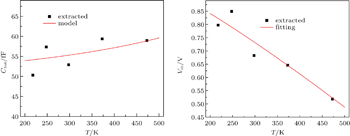Fig. 5. Temperature dependences of zero-bias capacitance and built-in voltage for BE junction.
 Figure Option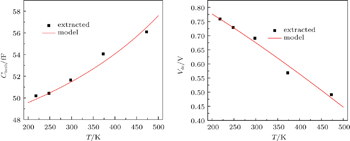Fig. 6. Temperature dependences of zero-bias capacitance and built-in voltage for BC junction.
 Figure Option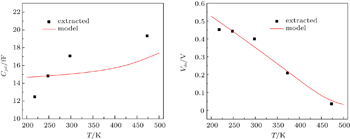Fig. 7. Temperature dependences of zero-bias capacitance and built-in voltage for CS junction.Table 1.

Extracted temperature coefficients for 1 × 0.2 × 16 μm2 SiGe HBT.

.

The temperature dependences of series resistances (RB, RCX, RE, and RSU) are shown in Fig. 8, from which good agreement is obtained over the whole measurement temperature range from 218 K to 473 K. Besides, it is found that the variation tendency of RE is different from those of the other three resistances as temperature increases. RB, RCX, and RSU gradually increase while RE decreases with temperature increasing, which may be due to the different scattering mechanisms contributing to carrier mobility.

 Figure Option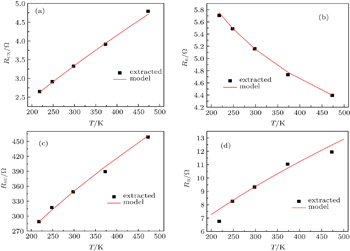Fig. 8. Temperature dependences of series resistances of (a) RCX, (b) RE, (c) RSU, and (d) RB.

Because of the low doping concentration, the carrier mobilities in base, collector and substrate region are mainly dominated by the lattice vibration scattering, which shows a temperature dependence of T3/2 and increases with temperature increasing. Therefore, the carrier mobility in these regions decreases and the corresponding series resistances increase as temperature increases. While in the emitter region, it is the ionized impurity scattering that dominates the carrier mobility due to the higher doping concentration (generally at a level of 1020 cm−3). The ionized impurity scattering shows a temperature dependence of T3/2 and hence decreases with temperature increasing; as a result, the carrier mobility in the emitter region increases and then the emitter resistance RE decreases.

5. Conclusions

In this paper, the temperature dependences of small-signal model parameters for SiGe HBT HICUM under cut-off mode are presented from 218 K to 473 K. The depletion capacitances are extracted from the cold S-parameter measurements. Emitter resistance RE is obtained from the forward-Gummel measurement. Base resistance RB is gained from cold S-parameters based on the rational function fitting. Then the temperature dependences of model parameters are determined based on the established temperature formulas. The proposed method is verified with a 1 × 0.2 × 16 μm2 SiGe HBT and good agreement is obtained over the whole temperature range. These results provide a theoretical guide for designing devices and circuits of SiGe HBTs in a wide temperature range.

Reference
 1 Sun Y BFu JXu JWang Y DZhou WZhang W 2014 Chin. Phys. 23 116104 2 Sun Y BFu JXu JWang Y DZhou WZhang W2013Acta Phys. Sin. 62196104(in Chinese) 3 Cressler J D 2010 IEEE Trans. Dev. Mater. Rel. 10 437 4 Cui YNiu G FShi YZhu C DNajafizadeh LCressler J DJoseph A2006IEEE Bipolar/BiCMOS Circuits Technol. Meeting1 5 Bhaskar BVenkataraman SYuan LLiang QLee CNuttinck SDekhyuon HChen YCressler J DLaskar JFreeman GAhlgren D C2005IEEE Trans. Electron Dev.4585 6 Chen TKuo W LZhao ELiang QJin ZCressler J DJoseph A J2004IEEE Trans. Electron Dev. 111825 7 Thomas D BNajafizadeh LCressler J DMoen K ALourenco N 2011 Solid-State Electron. 56 47 8 Yuan JCressler J DKrithivasan RThrivikraman TKhater M HAhlgren D CJoseph A JRieh J2009IEEE Trans. Electron Dev.561007 9 Cressler J DNiu G F2003Silicon-germanium heterojunction bipolar transistorsNorwoodArtech House 10 Schrer MChakravorty A2010Compact hierarchical modeling of bipolar transistors with HICUMSingaporeWorld Scientific 11 Raya CSchwartzmann TChevalier PPourchon FCeli DZimmer T2007IEEE Bipolar/BiCMOS Circuits Technol. Meeting188 12 Basaran UBerroth M2003IEEE Bipolar/BiCMOS Circuits Technol. Meeting93 13 Gabl RReisch M1998IEEE Trans. Electron Dev.122457 14 Yang JFu JSun Y BWang Y DZhou WZhang W2014IEEE International Conference on Electron Devices and Solid-State CircuitsChengdu, China1 15 Sun Y BFu JYang JWang Y DZhou WZhang W2014 12th IEEE International Conference on Solid-State and Integrated Circuit Technology Guilin, China 1 16 Schroter MMukherjee A2008HICUM-A Geometry Scalable Physics-Based Compact Bipolar Transistor Model Documentation of model level 2 version 2.23, University of Technology Dresden, Germany